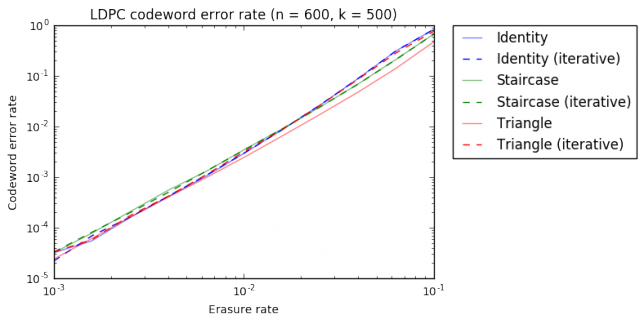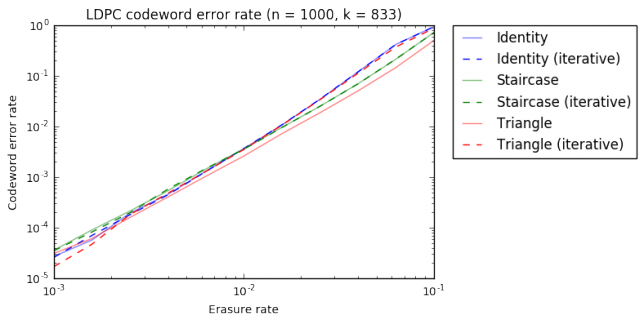# Testing LDPC code erasure decoding performance

In my previous post I talked about the RFC5170 LDPC codes used in Outernet. There I explained in some detail the pseudorandom construction of the LDPC codes and the simple erasure decoding algorithm used both in free-outernet and in the official closed-source receiver.

The Outernet LDPC codes follow what I call the “identity scheme”. This is different from the staircase and triangle schemes introduced in the RFC. The identity scheme already appeared in the literature, but it did not make it into the RFC. See, for instance, the report by Roca and Neumann Design, Evaluation and Comparison of Four Large Block FEC Codecs, LDPC, LDGM, LDGM Staircase and LDGM Triangle, plus a Reed-Solomon Small Block FEC Codec, especially Section 2, where it is called “LDGM”.

I also commented that erasure decoding for an LDPC code (or any other linear code) amounts to solving a linear system. This can be done using any algebraic method, such as Gaussian elimination. However, the simple decoding algorithm used in Outernet is as follows: try to find an equation with only one unknown, solve for that unknown, and repeat until the system is solved. Clearly this algorithm can fail even if the system can be solved (see my previous post for some examples and formal results). I will refer to this algorithm as iterative decoding, as it is done in the RFC.

With these two things in mind, I wondered about the performance of the LDPC codes used in Outernet and the iterative decoding algorithm. I’ve done some simulations and here I present my results.

The notation I use in this post is the same that I used in the previous post. I won’t introduce it again, so I refer the reader to the previous post to consult the notation.

I have done my simulations using Sage, since it provides a simple way of doing linear algebra over the field GF(2). I have used the Python code from free-outernet to generate the parity check matrices according to the RFC. To generate random numbers, I am using the most significant bits of the output of the Lehmer PRNG, as the RFC indicates, not the least significant bits (which are less random), as Outernet does. I guess that this difference doesn’t impact the results much.

To perform the simulations, I fix a value of $$n$$ and $$k$$. They are chosen to yield a rate $$k/n$$ close to $$5/6$$, as Outernet does. Then I generate the parity check matrix for the scheme to be tested (identity, staircase or triangle). Given a probability of erasure $$p$$, whether each of the symbols is erased or not is given by independent random variables distributed as a Bernoulli with parameter $$p$$. With this in mind, I generate the list of erased symbols randomly.

The naïve way to do this is, for each symbol, to generate a sample from a Bernoulli distribution with parameter $$p$$ and decide whether the symbol is erased or not. However, this algorithm needs a lot of random samples. There is a smarter way to do it which uses much fewer random samples when $$p$$ is small (which is the case in this kind of simulations). Note that the number $$e$$ of erased symbols is distributed as a Binomial of parameters $$n$$, $$p$$. We generate $$e$$ by taking a sample from this distribution. Then, we choose the $$e$$ erased symbols as follows: each of the symbols is chosen randomly from the set $$\{1,\ldots,n\}$$ (where each choice is equiprobable). If two of the erased symbols are chosen equal, then we choose again for the second one until we obtain a symbol that hasn’t been chosen already. These collisions are improbable when $$e$$ is small.

Given the list of erased symbols, we write the linear system $$Hr = 0$$ as $$Ax = b$$, where $$x$$ is the vector of unknowns corresponding to the erased symbols. Note that the matrix $$A$$ is obtained from $$H$$ by taking the columns corresponding to the erased symbols. As seen in the previous post, we know that the system $$Ax = b$$ has a solution. We are interested in whether it has a single solution, since erasure decoding is possible if and only if the solution is unique. This happens if and only if $$\ker A = 0$$, or equivalently, the $$\operatorname{rank} A = e$$. Finding the rank of the matrix $$A$$ over the field GF(2) is very easy to do with Sage.

To check if iterative decoding would succeed with this list of erasures, we look at the matrix $$A$$ again and proceed as follows. We look for a row of $$A$$ where only one entry is $$1$$. We remove that row and the column where the $$1$$ appeared. We continue in this manner until we get stuck or we obtain a matrix whose entries are all zeros. If we get stuck, iterative decoding is not possible. If we obtain the zero matrix, iterative decoding is possible. Note that this procedure mimics the iterative decoding algorithm, but we don’t explicitly solve for the unknowns, since we are not interested in their value.

For each value of $$p$$, we do $$10^6$$ trials, generating a list of erasures and checking whether erasure decoding is possible and whether iterative decoding would succeed. With this, we approximate the probability of decoding given an erasure rate $$p$$. I have simulated the cases $$n = 600$$, $$k=500$$ and $$n = 1000$$, $$k=833$$, for $$10^{-3} \leq p \leq 10^{-1}$$. These are typical values that one could see used in Outernet. The computations take many hours to run. The results are shown below.We observe the following. First, the relative performance of the six codes/algorithms studied is very similar in both graphs. Second, for the identity and staircase schemes, iterative decoding is as good as full algebraic decoding. This is a small surprise for me. However, for the triangle scheme, the difference between iterative decoding and algebraic decoding is significant. This is intuitive, since the identity and staircase matrices are rather sparse, but the triangle matrix has many ones.

The triangle scheme gives a much better performance than the other two schemes, but algebraic decoding is needed to take advantage of that improvement. If iterative decoding is done, the performance is actually worse.

Another interesting aspect is how the identity and staircase schemes compare. For high erasure rate $$p > 10^{-2}$$, the staircase scheme is better. However, for $$p < 10^{-2}$$ the identity scheme performs slightly better, and almost as well as the triangle scheme with algebraic decoding.

The Sage/Jupyter notebook used for the simulations in this post can be found here.

This site uses Akismet to reduce spam. Learn how your comment data is processed.